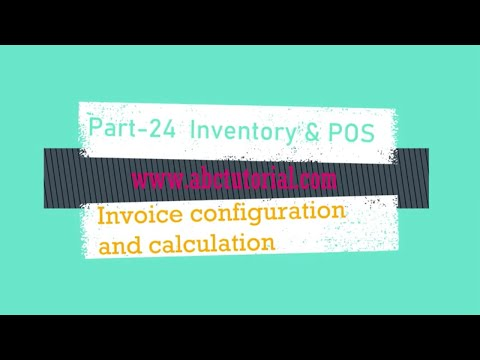## POS-24: Inventory Invoice Configuration and Calculation using JavaScript AngularJS ASP.NET MVC

• Teacher
• Category
• Tag
###### AngularJS , ASP.NET MVC , Jquery , Point of Sale(POS)
• Playlist

7/5/2021 1:17:38 AM

Post your any code related problem to www.abctutorial.com

Follow the previous video and articles to complete the POS tutorials

Step-1:

Write a method called SubTotalCalculation in HomeInvoiceController.js which will carry our subtotal calculation.

``````        \$scope.SubTotalCalculation = function () {
\$scope.Sale.Subtotal = 0;
for (var i = 0; i < \$scope.InvoiceCart.length; i++) {
\$scope.Sale.Subtotal = \$scope.Sale.Subtotal + \$scope.InvoiceCart[i].LineTotal;
}
}
``````

Step-2:

Put SubTotalCalculation() inside the ng-click of Product selection and Quantity input box.

• Product Selection selection box
``````<select ng-model="cart.ProductId" ng-click="SetValueOfProduct(cart.ProductId);SubTotalCalculation();">
<option>--Select--</option>
<option ng-repeat="product in ProductList" value="{{product.ProductId}}">{{product.Name}}</option>
</select>
``````
• Quantity Input box
``<input class="BorderLess" ng-model="cart.Quantity" ng-change="OnChangeLineTotalSet(cart.ProductId);SubTotalCalculation();" value="1" type="number" placeholder="Quantity" />``

Step-3:

Put the angularJs scope variable where you want to show the subtotal value

• Subtotal scope variable code.
``\${{Sale.Subtotal}}``

Step-4:

Now we want to show a dropdown box where we will select the Discount percentage then it will calculate

• Use this code to show Discount dropdown box
`````` <select ng-model="Sale.DiscountParcentage" ng-click="CalculateDiscount()">
<option value="">--Select--</option>
<option value="10">10%</option>
<option value="20">20%</option>
<option value="30">30%</option>
</select>
``````
• Use this code to show the calculated amount after discount
``\${{Sale.DiscountAmount}}``
`````` \$scope.CalculateDiscount = function () {
\$scope.Sale.DiscountAmount = (\$scope.Sale.Subtotal * \$scope.Sale.DiscountParcentage) / 100;
}
``````

Step-5:

Now we want to show a dropdown box where we will select the Vat percentage then it will calculate

• Use this code to show Vat dropdown box
`````` <select ng-model="Sale.VatParcentage" ng-click="CalculateVat()">
<option value="">--Select--</option>
<option value="10">10%</option>
<option value="20">20%</option>
<option value="30">30%</option>
</select>
``````
• Use this code to show the calculated amount after discount
``\${{Sale.VatAmount}}``
`````` \$scope.CalculateVat = function () {
\$scope.Sale.VatAmount = ((\$scope.Sale.Subtotal - \$scope.Sale.DiscountAmount) * \$scope.Sale.VatParcentage) / 100;
\$scope.Sale.TotalAmount = (\$scope.Sale.Subtotal - \$scope.Sale.DiscountAmount) + \$scope.Sale.VatAmount;
\$scope.Sale.TotalAmout = (\$scope.Sale.Subtotal - \$scope.Sale.DiscountAmount) + \$scope.Sale.VatAmount;
}
``````

Step-6:

Now we want to add a delete button which will allow us to delete the row clicking on the button.

``<a href="#" ng-click="AddNewRow()"><i class="fa fa-plus"></i></a>``
• Add this below javascript method to HomeInvoiceController.js which will delete the row if you click on delete button
``````         \$scope.RowDelete = function (index) {
if (index > -1) {
\$scope.InvoiceCart.splice(index, 1);
}
\$scope.SubTotalCalculation();
}
``````

Step-7:

Now run the project and see the output. If your code is not working then clear your cache.